# Population Growth Chapter 11 Geometric Growth Exponential Growth

• Slides: 18Population Growth Chapter 11 Geometric Growth Exponential Growth Logistic Population Growth Limits to Population Growth Density Dependent Density Independent Intrinsic Rates of Increase Our Future 1 1Geometric Growth • When generations do not overlap, growth can be modeled geometrically. Nt = Noλt Nt = Number of individuals at time t. v No = Initial number of individuals. v λ = Geometric rate of increase. v t = Number of time intervals or generations. v 2 2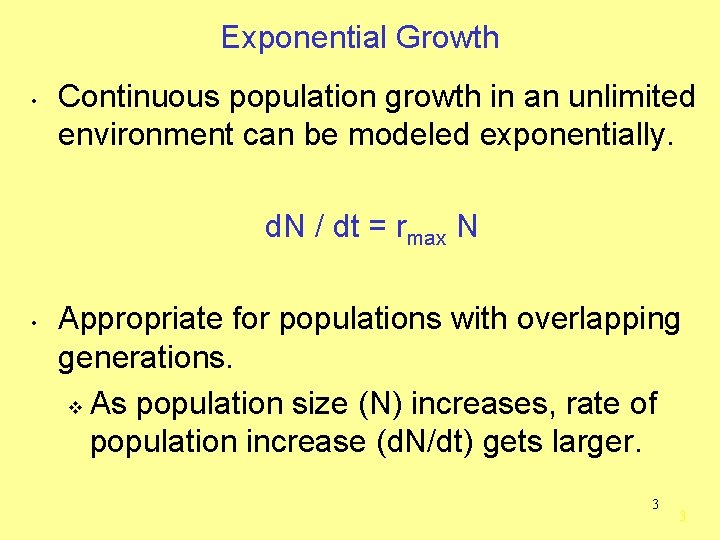Exponential Growth • Continuous population growth in an unlimited environment can be modeled exponentially. d. N / dt = rmax N • Appropriate for populations with overlapping generations. v As population size (N) increases, rate of population increase (d. N/dt) gets larger. 3 3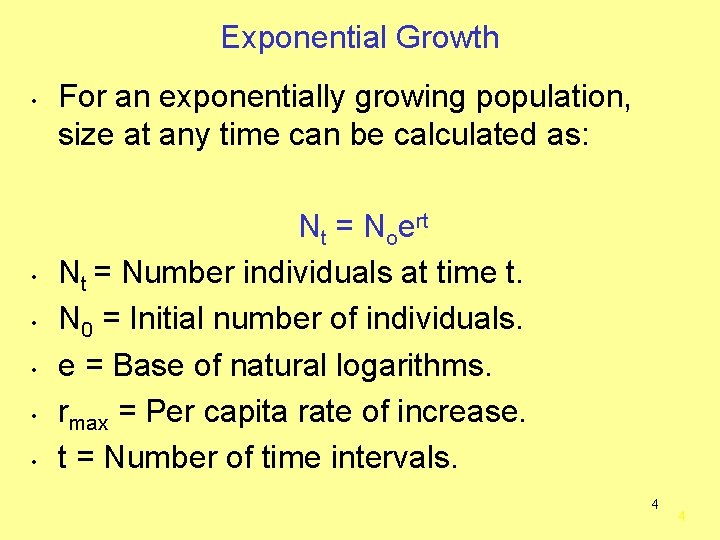Exponential Growth • • • For an exponentially growing population, size at any time can be calculated as: Nt = Noert Nt = Number individuals at time t. N 0 = Initial number of individuals. e = Base of natural logarithms. rmax = Per capita rate of increase. t = Number of time intervals. 4 4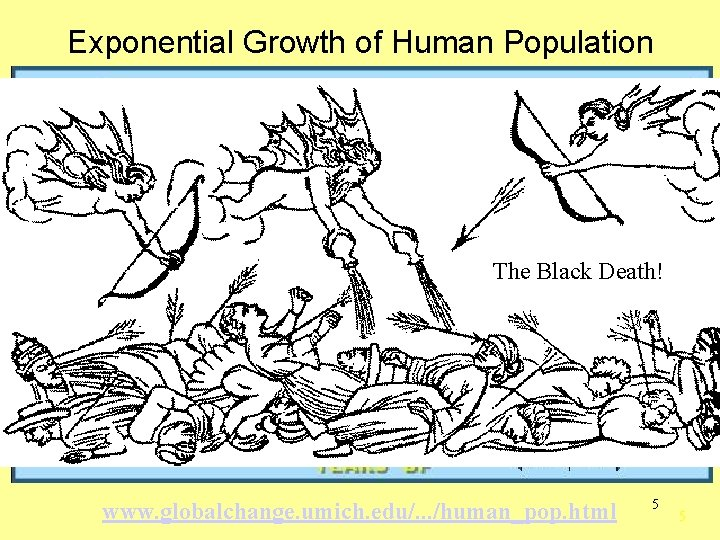Exponential Growth of Human Population The Black Death! www. globalchange. umich. edu/. . . /human_pop. html 5 5Logistic Population Growth • As resources are depleted, population growth rate slows and eventually stops, this is called logistic population growth. v Sigmoid (S-shaped) pop. growth curve. v Carrying capacity (K) is the number of individuals of a population the environment can support. § A finite amount of resources can only support a finite number of individuals. 6 6Logistic Population Growth 7 7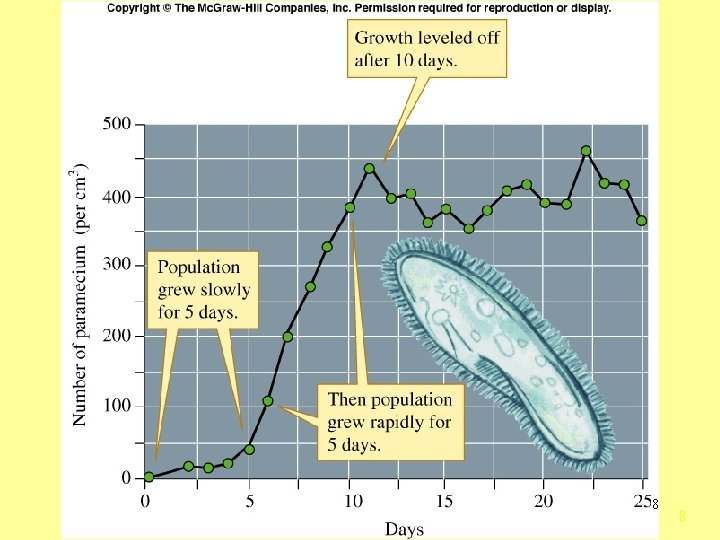8 8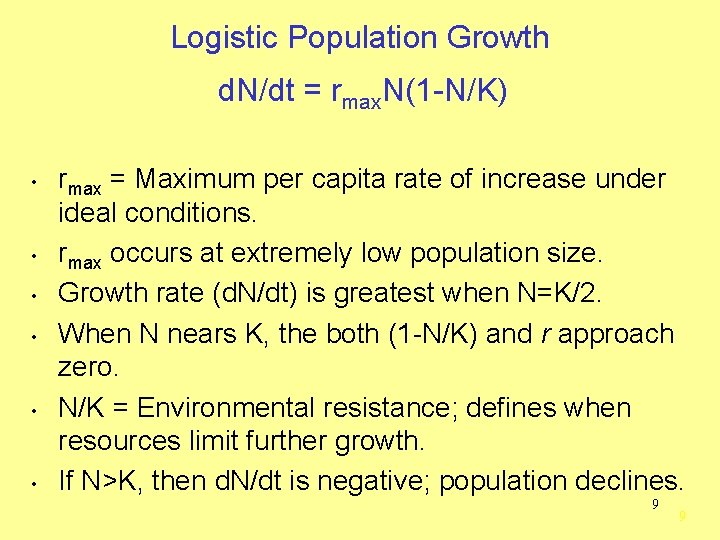Logistic Population Growth d. N/dt = rmax. N(1 -N/K) • • • rmax = Maximum per capita rate of increase under ideal conditions. rmax occurs at extremely low population size. Growth rate (d. N/dt) is greatest when N=K/2. When N nears K, the both (1 -N/K) and r approach zero. N/K = Environmental resistance; defines when resources limit further growth. If N>K, then d. N/dt is negative; population declines. 9 9Limits to Population Growth • Environment limits population growth by altering birth and death rates. v Density-dependent factors § Disease, Resource competition v Density-independent factors § Natural disasters 10 10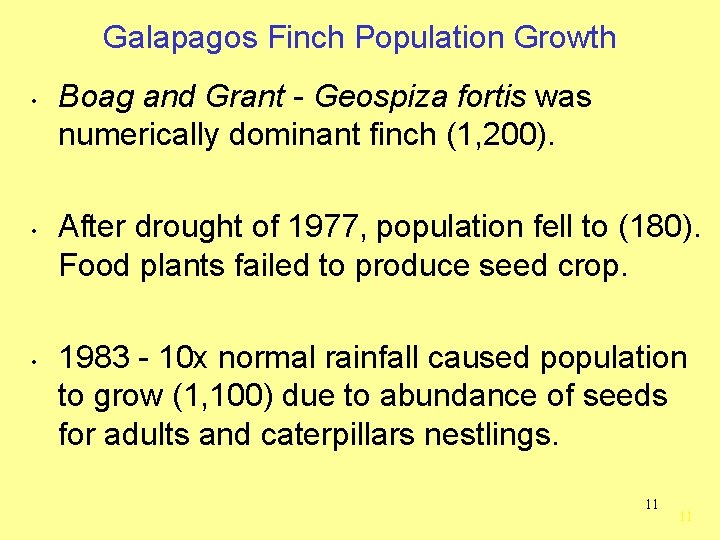Galapagos Finch Population Growth • • • Boag and Grant - Geospiza fortis was numerically dominant finch (1, 200). After drought of 1977, population fell to (180). Food plants failed to produce seed crop. 1983 - 10 x normal rainfall caused population to grow (1, 100) due to abundance of seeds for adults and caterpillars nestlings. 11 11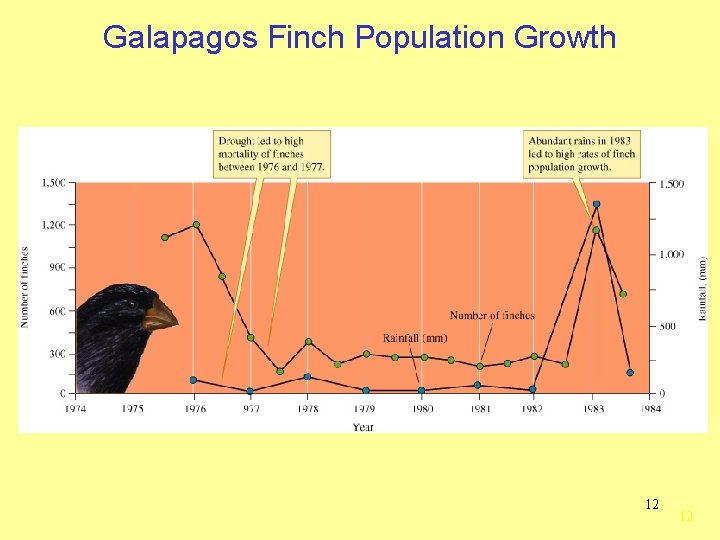Galapagos Finch Population Growth 12 12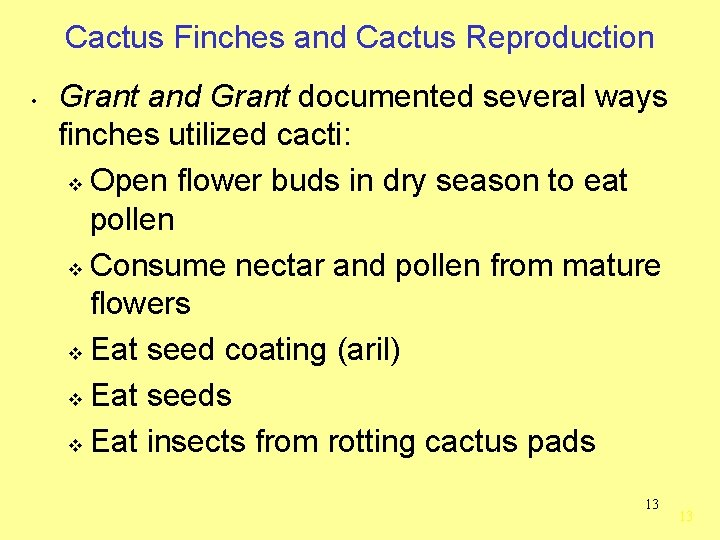Cactus Finches and Cactus Reproduction • Grant and Grant documented several ways finches utilized cacti: v Open flower buds in dry season to eat pollen v Consume nectar and pollen from mature flowers v Eat seed coating (aril) v Eat seeds v Eat insects from rotting cactus pads 13 13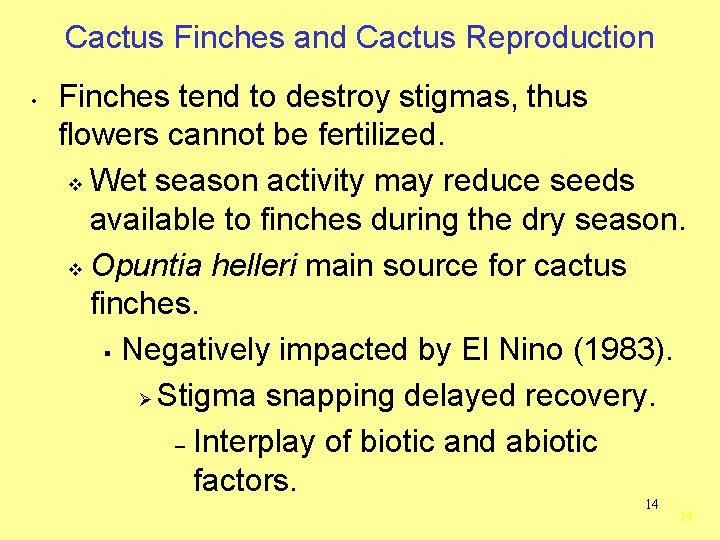Cactus Finches and Cactus Reproduction • Finches tend to destroy stigmas, thus flowers cannot be fertilized. v Wet season activity may reduce seeds available to finches during the dry season. v Opuntia helleri main source for cactus finches. § Negatively impacted by El Nino (1983). Ø Stigma snapping delayed recovery. – Interplay of biotic and abiotic factors. 14 14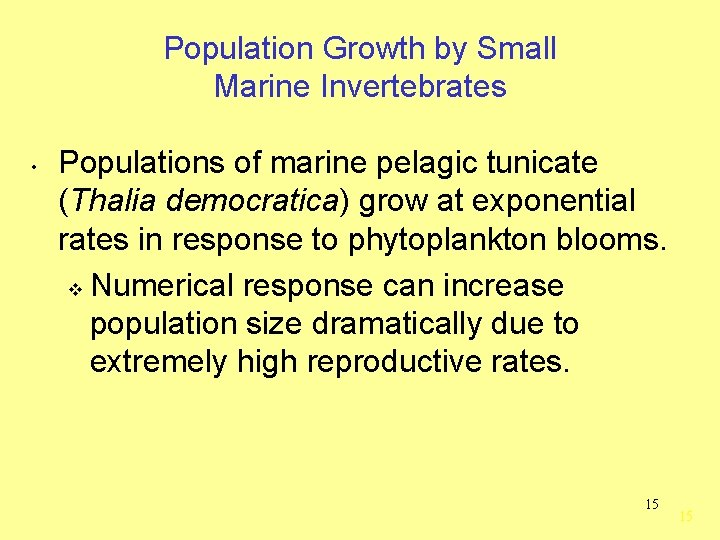Population Growth by Small Marine Invertebrates • Populations of marine pelagic tunicate (Thalia democratica) grow at exponential rates in response to phytoplankton blooms. v Numerical response can increase population size dramatically due to extremely high reproductive rates. 15 15Intrinsic Rates of Increase (r) • On average, small organisms have higher rates of per capita increase and more variable populations than large organisms. 16 16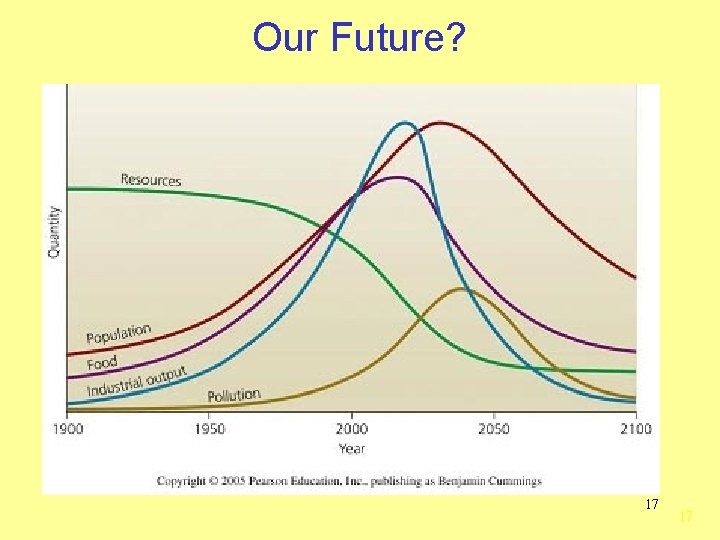Our Future? 17 1718 18